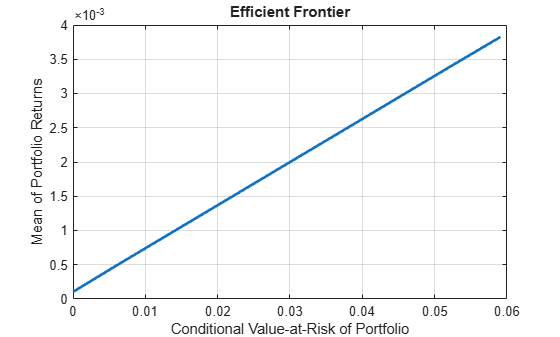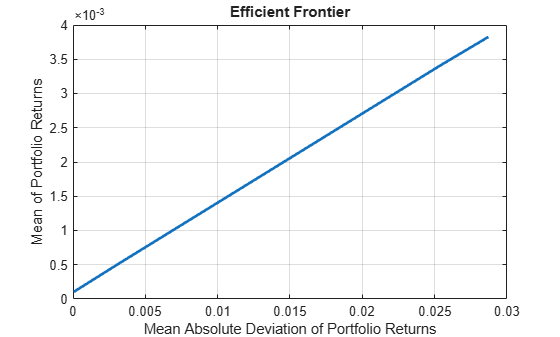# simulateNormalScenariosByData

Simulate multivariate normal asset return scenarios from data

Using a `fints` object for the `AssetReturns` argument of `simulateNormalScenariosByData` is not recommended. Use `timetable` instead for financial time series. For more information, see Convert Financial Time Series Objects fints to Timetables.

## Syntax

``obj = simulateNormalScenariosByData(obj,AssetReturns)``
``obj = simulateNormalScenariosByData(obj,AssetReturns,NumScenarios,Name,Value)``

## Description

example

````obj = simulateNormalScenariosByData(obj,AssetReturns)` simulates multivariate normal asset return scenarios from data for portfolio object for `PortfolioCVaR` or `PortfolioMAD` objects. For details on the workflows, see PortfolioCVaR Object Workflow, and PortfolioMAD Object Workflow.```

example

````obj = simulateNormalScenariosByData(obj,AssetReturns,NumScenarios,Name,Value)` simulates multivariate normal asset return scenarios from data for portfolio object for `PortfolioCVaR` or `PortfolioMAD` objects using additional options specified by one or more `Name,Value` pair arguments.This function estimates the mean and covariance of asset returns from either price or return data and then uses these estimates to generate the specified number of scenarios with the function `mvnrnd`. Data can be in a `NumSamples`-by-`NumAssets` matrix of `NumSamples` prices or returns at a given periodicity for a collection of `NumAssets` assets, a `table` or a `timetable`. NoteIf you want to use the method multiple times and you want to simulate identical scenarios each time the function is called, precede each function call with `rng`(seed) using a specified integer seed. ```

## Examples

collapse all

Given a PortfolioCVaR object `p`, use the `simulateNormalScenariosByData` function to simulate multivariate normal asset return scenarios from data.

```m = [ 0.05; 0.1; 0.12; 0.18 ]; C = [ 0.0064 0.00408 0.00192 0; 0.00408 0.0289 0.0204 0.0119; 0.00192 0.0204 0.0576 0.0336; 0 0.0119 0.0336 0.1225 ]; m = m/12; C = C/12; RawData = mvnrnd(m, C, 240); NumScenarios = 2000; p = PortfolioCVaR; p = simulateNormalScenariosByData(p, RawData, NumScenarios)```
```p = PortfolioCVaR with properties: BuyCost: [] SellCost: [] RiskFreeRate: [] ProbabilityLevel: [] Turnover: [] BuyTurnover: [] SellTurnover: [] NumScenarios: 2000 Name: [] NumAssets: 4 AssetList: [] InitPort: [] AInequality: [] bInequality: [] AEquality: [] bEquality: [] LowerBound: [] UpperBound: [] LowerBudget: [] UpperBudget: [] GroupMatrix: [] LowerGroup: [] UpperGroup: [] GroupA: [] GroupB: [] LowerRatio: [] UpperRatio: [] MinNumAssets: [] MaxNumAssets: [] BoundType: [] ```
```p = setDefaultConstraints(p); p = setProbabilityLevel(p, 0.9); disp(p);```
``` PortfolioCVaR with properties: BuyCost: [] SellCost: [] RiskFreeRate: [] ProbabilityLevel: 0.9000 Turnover: [] BuyTurnover: [] SellTurnover: [] NumScenarios: 2000 Name: [] NumAssets: 4 AssetList: [] InitPort: [] AInequality: [] bInequality: [] AEquality: [] bEquality: [] LowerBound: [4x1 double] UpperBound: [] LowerBudget: 1 UpperBudget: 1 GroupMatrix: [] LowerGroup: [] UpperGroup: [] GroupA: [] GroupB: [] LowerRatio: [] UpperRatio: [] MinNumAssets: [] MaxNumAssets: [] BoundType: [4x1 categorical] ```

To illustrate using the `simulateNormalScenariosByData` function with `AssetReturns` data contined in a `timetable` object, use the `CAPMuniverse.mat` which contains a `timetable` object (`AssetTimeTable`) for returns data.

```load CAPMuniverse; AssetsTimeTable.Properties```
```ans = TimetableProperties with properties: Description: '' UserData: [] DimensionNames: {'Time' 'Variables'} VariableNames: {1x14 cell} VariableDescriptions: {} VariableUnits: {} VariableContinuity: [] RowTimes: [1471x1 datetime] StartTime: 03-Jan-2000 SampleRate: NaN TimeStep: NaN CustomProperties: No custom properties are set. Use addprop and rmprop to modify CustomProperties. ```
`head(AssetsTimeTable,5)`
``` Time AAPL AMZN CSCO DELL EBAY GOOG HPQ IBM INTC MSFT ORCL YHOO MARKET CASH ___________ _________ _________ _________ _________ _________ ____ _________ _________ _________ _________ _________ _________ _________ __________ 03-Jan-2000 0.088805 0.1742 0.008775 -0.002353 0.12829 NaN 0.03244 0.075368 0.05698 -0.001627 0.054078 0.097784 -0.012143 0.00020522 04-Jan-2000 -0.084331 -0.08324 -0.05608 -0.08353 -0.093805 NaN -0.075613 -0.033966 -0.046667 -0.033802 -0.0883 -0.067368 -0.03166 0.00020339 05-Jan-2000 0.014634 -0.14877 -0.003039 0.070984 0.066875 NaN -0.006356 0.03516 0.008199 0.010567 -0.052837 -0.073363 0.011443 0.00020376 06-Jan-2000 -0.086538 -0.060072 -0.016619 -0.038847 -0.012302 NaN -0.063688 -0.017241 -0.05824 -0.033477 -0.058824 -0.10307 0.011743 0.00020266 07-Jan-2000 0.047368 0.061013 0.0587 -0.037708 -0.000964 NaN 0.028416 -0.004386 0.04127 0.013091 0.076771 0.10609 0.02393 0.00020157 ```

Notice that GOOG has missing data (`NaN`) becuse it was not listed before Aug 2004. The `simulateNormalScenariosByData` function has a name-value pair argument `'MissingData'` that indicates with a Boolean value whether to use the missing data capabilities of Financial Toolbox™ software. The default value for 'MissingData' is `false` which removes all samples with `NaN` values. If, however, `'MissingData'` is set to `true`, the `estimateAssetMoments` function uses the ECM algorithm to estimate asset moments. `The simulateNormalScenariosByData `function also accepts a name-value pair argument `'DataFormat'` with a corresponding value set to `'prices'` to indicate that the input to the function is in the form of asset prices and not returns (the default value for the `'DataFormat'` argument is `'returns'`).

```NumScenarios = 100; r = PortfolioCVaR; r = simulateNormalScenariosByData(r,AssetsTimeTable,NumScenarios,'DataFormat','Returns','MissingData',true);```

In addition, `simulateNormalScenariosByData `extracts asset names or identifiers from a `timetable` object when the name-value argument `'GetAssetList'` is set to `true` (its default value is `false`). If the `'GetAssetList'` value is `true`, the timetable column identifiers are used to set the `AssetList` property of the `PortfolioCVaR` object. To show this, the formation of the `PortfolioCVaR` object `r` is repeated with the `'GetAssetList'` flag set to `true.`

```r = simulateNormalScenariosByData(r,AssetsTimeTable,NumScenarios,'GetAssetList',true); disp(r.AssetList)```
``` Columns 1 through 6 {'AAPL'} {'AMZN'} {'CSCO'} {'DELL'} {'EBAY'} {'GOOG'} Columns 7 through 12 {'HPQ'} {'IBM'} {'INTC'} {'MSFT'} {'ORCL'} {'YHOO'} Columns 13 through 14 {'MARKET'} {'CASH'} ```

Create a PortfolioCVaR object `p` and use the `simulateNormalScenariosByData` function with market data loaded from `CAPMuniverse.mat` to simulate multivariate normal asset return scenarios. The market data, `AssetsTimeTable`, is a `timetable` of asset returns.

```load CAPMuniverse p = PortfolioCVaR('AssetList',Assets); disp(p);```
``` PortfolioCVaR with properties: BuyCost: [] SellCost: [] RiskFreeRate: [] ProbabilityLevel: [] Turnover: [] BuyTurnover: [] SellTurnover: [] NumScenarios: [] Name: [] NumAssets: 14 AssetList: {1x14 cell} InitPort: [] AInequality: [] bInequality: [] AEquality: [] bEquality: [] LowerBound: [] UpperBound: [] LowerBudget: [] UpperBudget: [] GroupMatrix: [] LowerGroup: [] UpperGroup: [] GroupA: [] GroupB: [] LowerRatio: [] UpperRatio: [] MinNumAssets: [] MaxNumAssets: [] BoundType: [] ```

Simulate the scenarios from the timetable data for each of the assets from `CAPMuniverse.mat `and plot the efficient frontier.

```p = simulateNormalScenariosByData(p,AssetsTimeTable,10000,'missingdata',true); p = setDefaultConstraints(p); p = setProbabilityLevel(p, 0.9); plotFrontier(p);```Given a PortfolioMAD object `p`, use the `simulateNormalScenariosByData` function to simulate multivariate normal asset return scenarios from data.

```m = [ 0.05; 0.1; 0.12; 0.18 ]; C = [ 0.0064 0.00408 0.00192 0; 0.00408 0.0289 0.0204 0.0119; 0.00192 0.0204 0.0576 0.0336; 0 0.0119 0.0336 0.1225 ]; m = m/12; C = C/12; RawData = mvnrnd(m, C, 240); NumScenarios = 2000; p = PortfolioMAD; p = simulateNormalScenariosByData(p, RawData, NumScenarios); p = setDefaultConstraints(p); disp(p);```
``` PortfolioMAD with properties: BuyCost: [] SellCost: [] RiskFreeRate: [] Turnover: [] BuyTurnover: [] SellTurnover: [] NumScenarios: 2000 Name: [] NumAssets: 4 AssetList: [] InitPort: [] AInequality: [] bInequality: [] AEquality: [] bEquality: [] LowerBound: [4x1 double] UpperBound: [] LowerBudget: 1 UpperBudget: 1 GroupMatrix: [] LowerGroup: [] UpperGroup: [] GroupA: [] GroupB: [] LowerRatio: [] UpperRatio: [] MinNumAssets: [] MaxNumAssets: [] BoundType: [4x1 categorical] ```

Create a PortfolioMAD object `p` and use the `simulateNormalScenariosByData` function with market data loaded from `CAPMuniverse.mat` to simulate multivariate normal asset return scenarios. The market data, `AssetsTimeTable`, is a `timetable` of asset returns.

```load CAPMuniverse p = PortfolioMAD('AssetList',Assets); disp(p.AssetList');```
``` {'AAPL' } {'AMZN' } {'CSCO' } {'DELL' } {'EBAY' } {'GOOG' } {'HPQ' } {'IBM' } {'INTC' } {'MSFT' } {'ORCL' } {'YHOO' } {'MARKET'} {'CASH' } ```

Simulate the scenarios from the timetable data for each of the assets from `CAPMuniverse.mat `and plot the efficient frontier.

```p = simulateNormalScenariosByData(p,AssetsTimeTable,10000,'missingdata',true); p = setDefaultConstraints(p); plotFrontier(p);```## Input Arguments

collapse all

Object for portfolio, specified using a `PortfolioCVaR` or `PortfolioMAD` object.

For more information on creating a `PortfolioCVaR` or `PortfolioMAD` object, see

Data Types: `object`

Asset data that can be converted into asset returns ([`NumSamples`-by-`NumAssets`] matrix), specified as a matrix, `table`, or `timetable`.

`AssetReturns` data can be:

• `NumSamples`-by-`NumAssets` matrix.

• Table of `NumSamples` prices or returns at a given periodicity for a collection of `NumAssets` assets

• Timetable object with `NumSamples` observations and `NumAssets` time series

Data Types: `double` | `table` | `timetable`

Number of scenarios to simulate, specified as a positive integer.

Data Types: `double`

### Name-Value Arguments

Specify optional pairs of arguments as `Name1=Value1,...,NameN=ValueN`, where `Name` is the argument name and `Value` is the corresponding value. Name-value arguments must appear after other arguments, but the order of the pairs does not matter.

Before R2021a, use commas to separate each name and value, and enclose `Name` in quotes.

Example: ```p = simulateNormalScenariosByData(p,RawData,NumScenarios,'DataFormat','Returns','MissingData',true,'GetAssetList',true)```

Flag to convert input data as prices into returns, specified as the comma-separated pair consisting of `'DataFormat'` and a character vector with the values:

• `'Returns'` — Data in `AssetReturns` contains asset total returns.

• `'Prices'` — Data in `AssetReturns` contains asset total return prices.

Data Types: `char`

Flag to use ECM algorithm to handle `NaN` values, specified as the comma-separated pair consisting of `'MissingData'` and a logical with a value of `true` or `false`.

• `false` — Do not use ECM algorithm to handle `NaN` values (exclude `NaN` values).

• `true` — Use ECM algorithm to handle `NaN` values.

Data Types: `logical`

Flag indicating which asset names to use for the asset list, specified as the comma-separated pair consisting of `'GetAssetList'` and a logical with a value of `true` or `false`.

• `false` — Do not extract or create asset names.

• `true` — Extract or create asset names from the table or timetable.

If a `table` or `timetable` is passed into this function using the `AssetReturns` argument and the `GetAssetList` flag is `true`, the column names from the `table` or `timetable` are used as asset names in `obj.AssetList`.

If a matrix is passed and the `GetAssetList` flag is `true`, default asset names are created based on the `AbstractPortfolio` property `defaultforAssetList`, which is `'Asset'`.

If the `GetAssetList` flag is `false`, no action occurs, which is the default behavior.

Data Types: `logical`

## Output Arguments

collapse all

Updated portfolio object, returned as a `PortfolioCVaR` or `PortfolioMAD` object. For more information on creating a portfolio object, see

## Tips

You can also use dot notation to simulate multivariate normal asset return scenarios from data for a PortfolioCVaR or PortfolioMAD object.

`obj = obj.simulateNormalScenariosByData(AssetReturns,NumScenarios,Name,Value);`

## Version History

Introduced in R2012b